# An estimated force-time curve for a baseball struck by a bat is shown in the figure....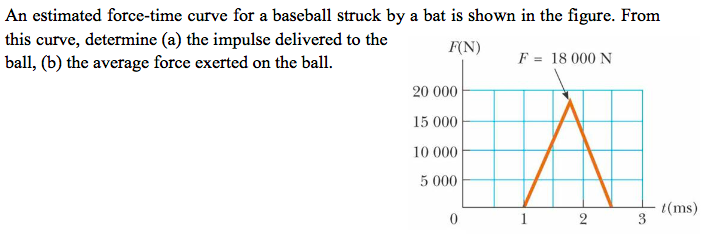An estimated force-time curve for a baseball struck by a bat is shown in the figure. From this curve, determine (a) the impulse delivered to the ball, (b) the average force exerted on the ball FN) F- 18 000 N 20 000 15 000 10 000 5000 t (ms)

Impulse delivered

I = area under Ft curve

I = 0.5* (0.0025 - 0.001)* 18000 = 13.5 N.s

======

Force = impulse / t = 13.5/0.0015 = 9000 N

##### Add Answer to: An estimated force-time curve for a baseball struck by a bat is shown in the figure....
Similar Homework Help Questions
• ### An estimated force-time curve for a baseball struck by a bat is shown in the figure below. Let Fmax = 15,000 N, ta = 0....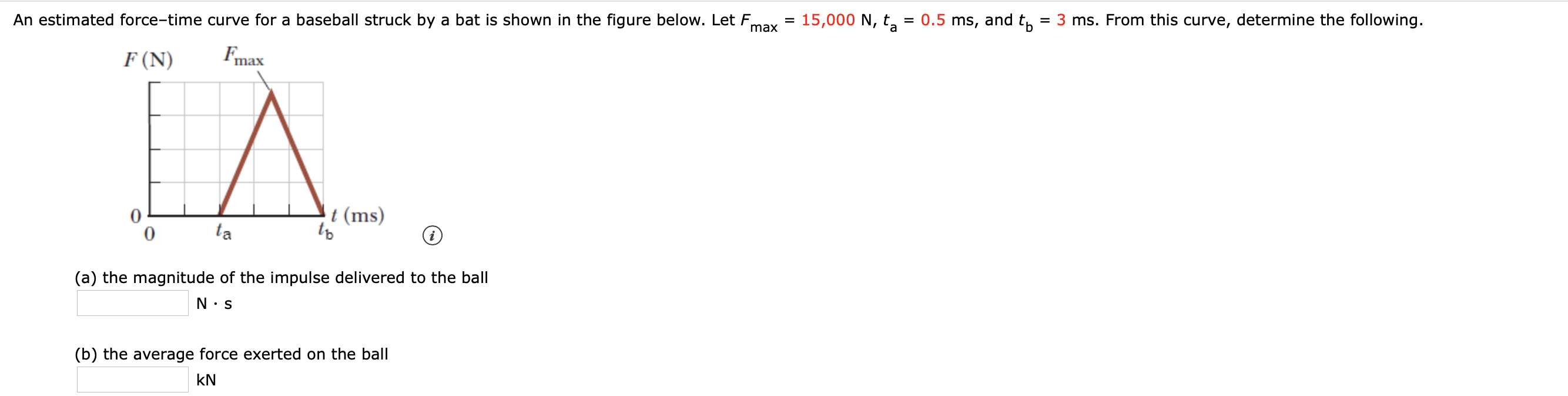An estimated force-time curve for a baseball struck by a bat is shown in the figure below. Let Fmax = 15,000 N, ta = 0.5 ms, and to = 3 ms. From this curve, determine the following. F(N) max (a) the magnitude of the impulse delivered to the ball I.S (b) the average force exerted on the ball KN

• ### 8. An estimated force vs. time curve for a baseball struck by a bat is shown in Figure P6.8....

8. An estimated force vs. time curve for a baseball struck by a bat is shown in Figure P6.8. From this curve, determine (a) the impulse delivered to the ball and (b)the average force exerted on the ball.

• ### physics problem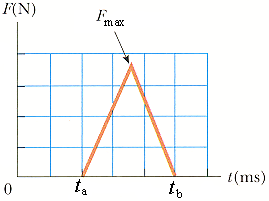An estimated force–time curve for a baseball struck by a bat is shown in the figure below. LetFmax = 19 000 N, ta = 1.5 ms, and tb = 3 ms.From this curve, determine each of the following.(a) the magnitude of the impulse delivered to the ballN · s(b) the average force exerted on the ballkN

• ### Average forceAn estimated force-time curve for a baseball struck by a bat isshown in the figure below. Let Fmax =15000 N, ta =0.5 ms, andtb =3 ms.From this curve, determine each of the following.(a) the impulse delivered to the ball1 N·s(b) the average force exerted on the ball2 N(c) the peak force exerted on the ball3 N

• ### questionsAn estimated force-time curvefor a baseball struck by a bat is shown. Let Fmax= 17000N, ta= 0.5 ms and tb= 2.5ms.From this curve, determine:(a)the impulse delivered to the ball(b)the average force exerted on the ball(c)the peak force exerted on the ball

• ### A 0.245 kg baseball is struck by a bat. Just before impact, the ball is traveling...

A 0.245 kg baseball is struck by a bat. Just before impact, the ball is traveling horizontally to the right at 40.0m/s, and leaves the bat traveling to the left at an angle 30.0º above the horizontal with a speed of 60.0m/s. a) Determine the Impulse on the ball. b) If the ball and bat are in contact for 3.50ms, determine the average force exerted on the ball.

• ### .1 T-Mobile LTE 8:19 PM 14% AA webassign.net 6. 010 points Previous Answers SerCP116.1.P.002. My Notes...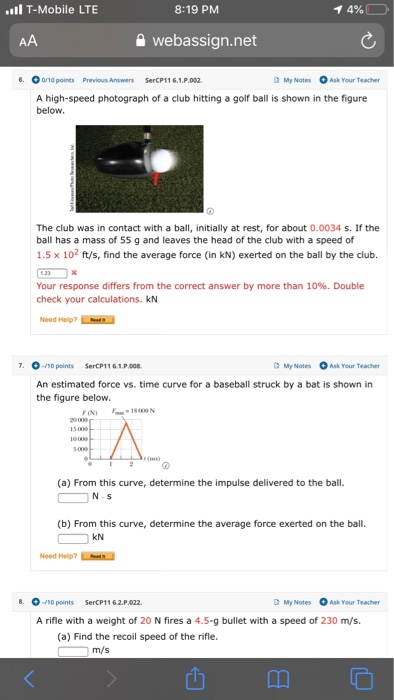.1 T-Mobile LTE 8:19 PM 14% AA webassign.net 6. 010 points Previous Answers SerCP116.1.P.002. My Notes Ask Your Teacher A high-speed photograph of a club hitting a golf ball is shown in the figure below. The club was in contact with a ball, initially at rest, for about 0.0034 s. If the ball has a mass of 55 g and leaves the head of the club with a speed of 1.5 x 102 ft/s, find the average force (in kN)...

• ### The impulse shown is applied to a 2.0 kg object. If the object is initially at...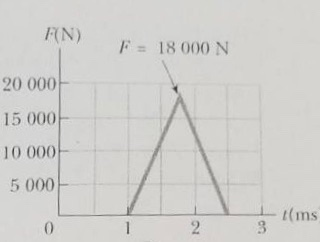The impulse shown is applied to a 2.0 kg object. If the object is initially at rest, what is the velocity after the force is applied? FN)F 18 000 N 20 000 15 000 10 000 5 000 t(ms

• ### A baseball (m-0.1 kg) has an initial velocity of-30m/s as it approaches a bat. We have...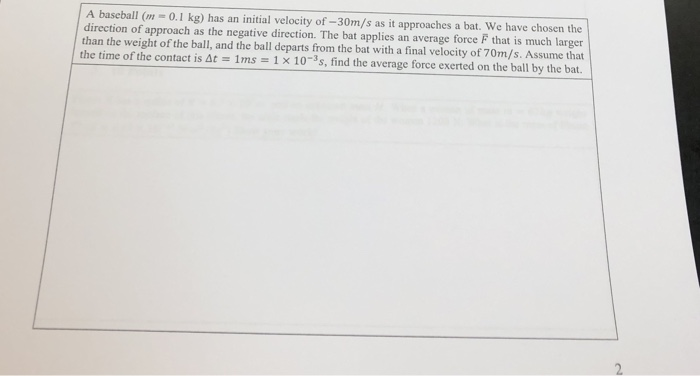A baseball (m-0.1 kg) has an initial velocity of-30m/s as it approaches a bat. We have chosen the direction of approach as the negative direction. The bat applies an average force F that is much larger than the weight of the ball, and the ball departs from the bat with a final velocity of 70m/s. Assume that the time of the contact is At 1 ms-1 x 10-35, find the average force exerted on the ball by the bat. 2

• ### A 156g baseball is thrown with a speed of 22 m/s. It is hit in a...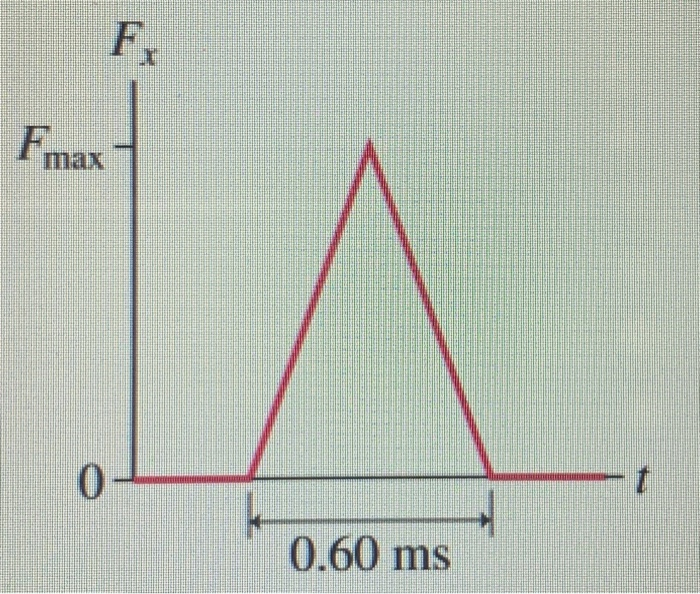A 156g baseball is thrown with a speed of 22 m/s. It is hit in a straight line back toward the pitcher at a speed of 37 m/s. The impulse force of the bat is shown. (a) What is the maximum force Fmax that the bat exerts on the ball? (b) What is the average force of the bat on the ball? Fx F max t 0 0.60 ms

Free Homework App